### Trigonometry

Trigonometry starts with something remarkably simple: comparing the measurements on sides of right triangles, like the one below: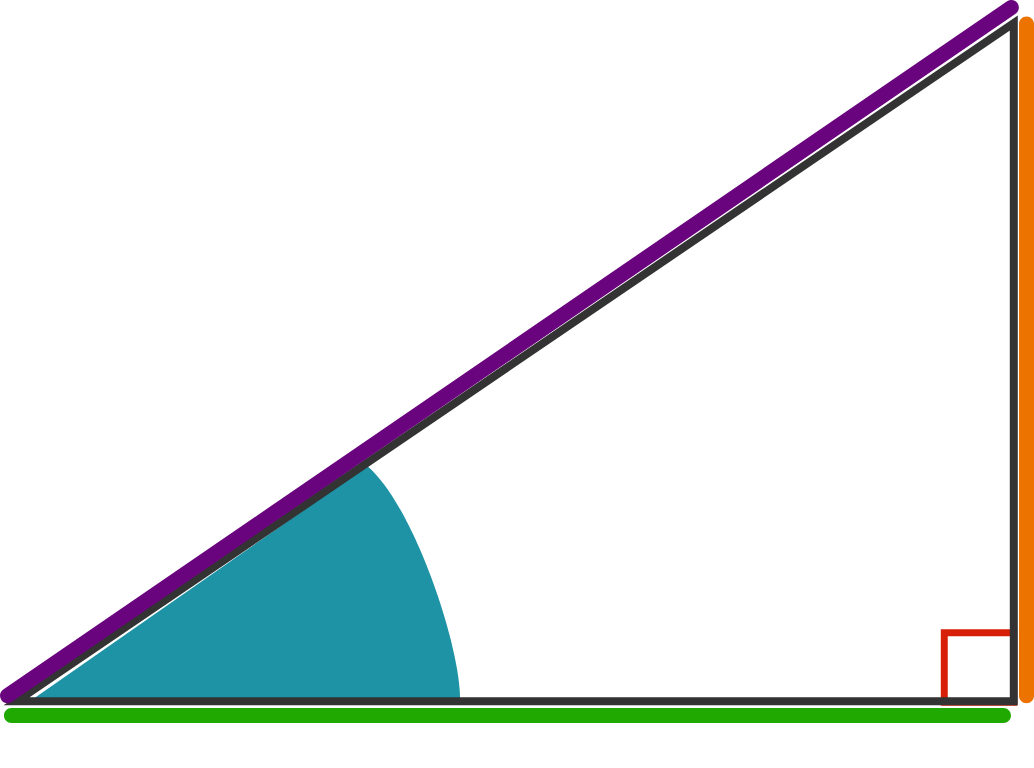From this beginning, it extracts the functions of sine, cosine, and tangent and builds one of the most versatile structures in mathematics! While they get used in "measurement" problems like finding the lengths of sides in geometric figures and on maps, they also link in a fuller sense to some of the most fundamental things in the universe, like springs, pendulums, light, and sound!

# Exploring the Sine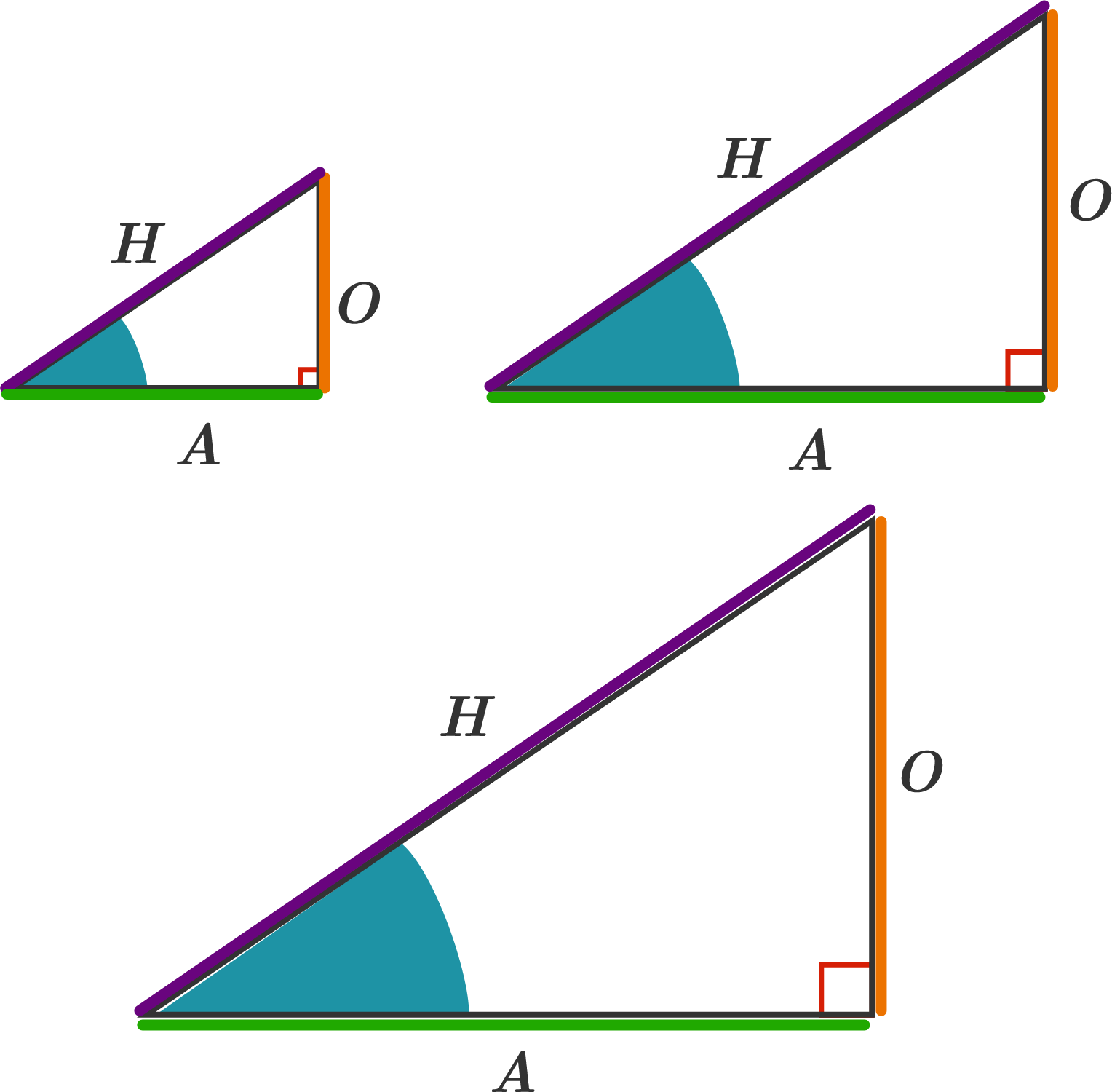The triangles above are all similar (same shape, different sizes).

Which of these ratios never changes, no matter the size of triangle?

# Exploring the SineWe can define the sine of an angle in a right triangle as the length of the side opposite to the angle (marked orange) divided by the hypotenuse (marked purple). We can reduce in symbols using the diagram above to say $\sin(x) = \frac{O}{H} .$

If all the marked angles in the right triangles below are congruent, what is the value of $Q?$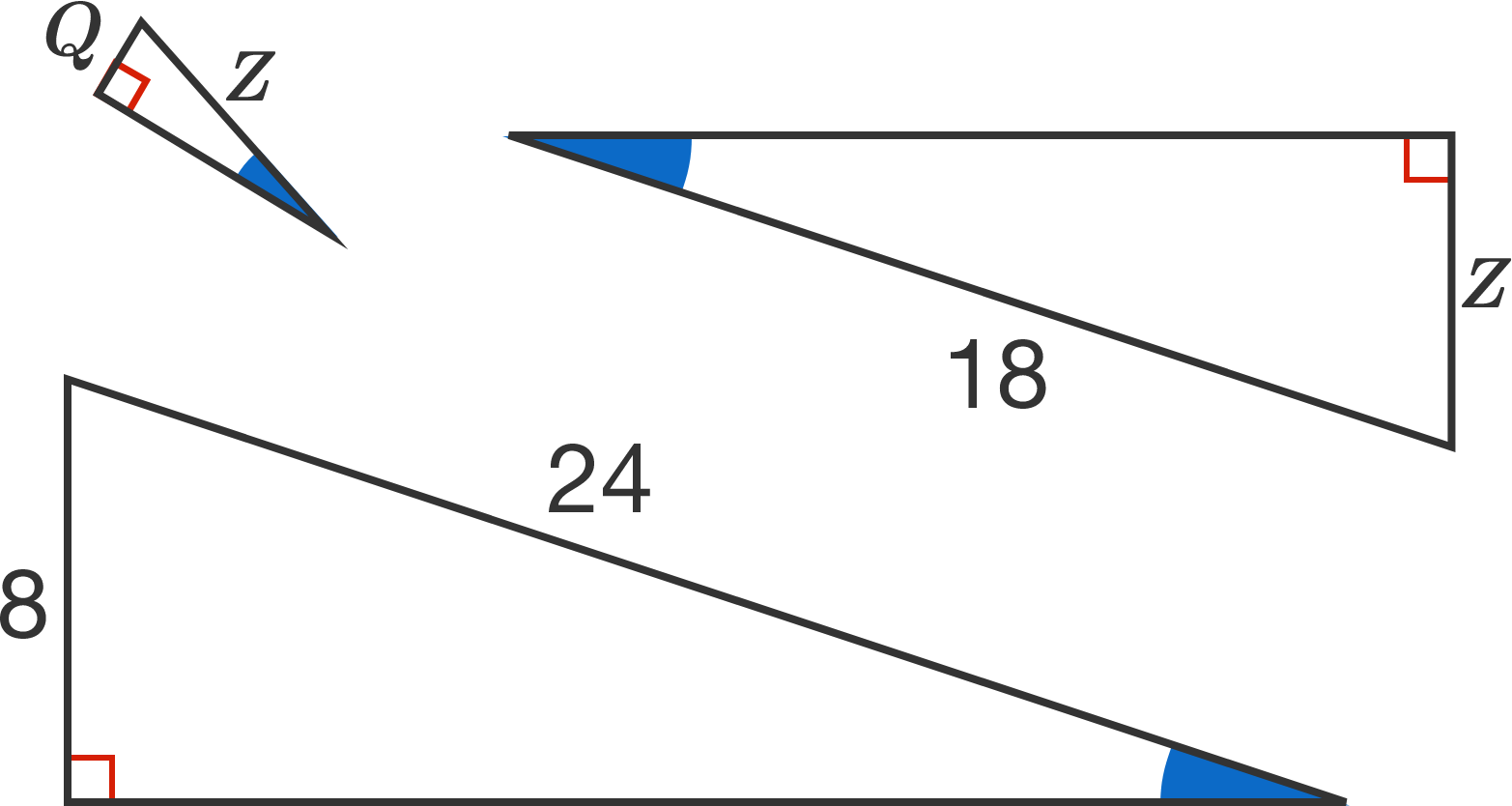# Exploring the Sine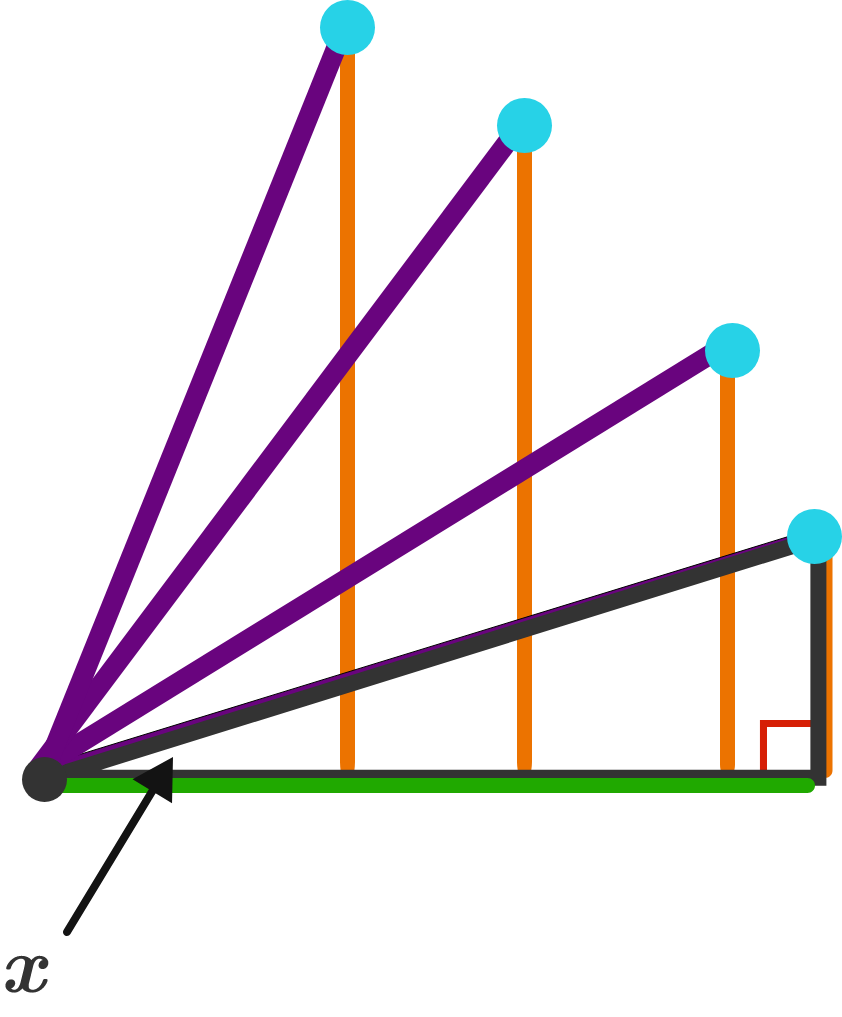Imagine now that you keep track of the marked blue point as the angle $x$ increases such that the hypotenuse of the triangle remains a constant length. Part of what kind of shape is traced by the blue point?

# Exploring the Sine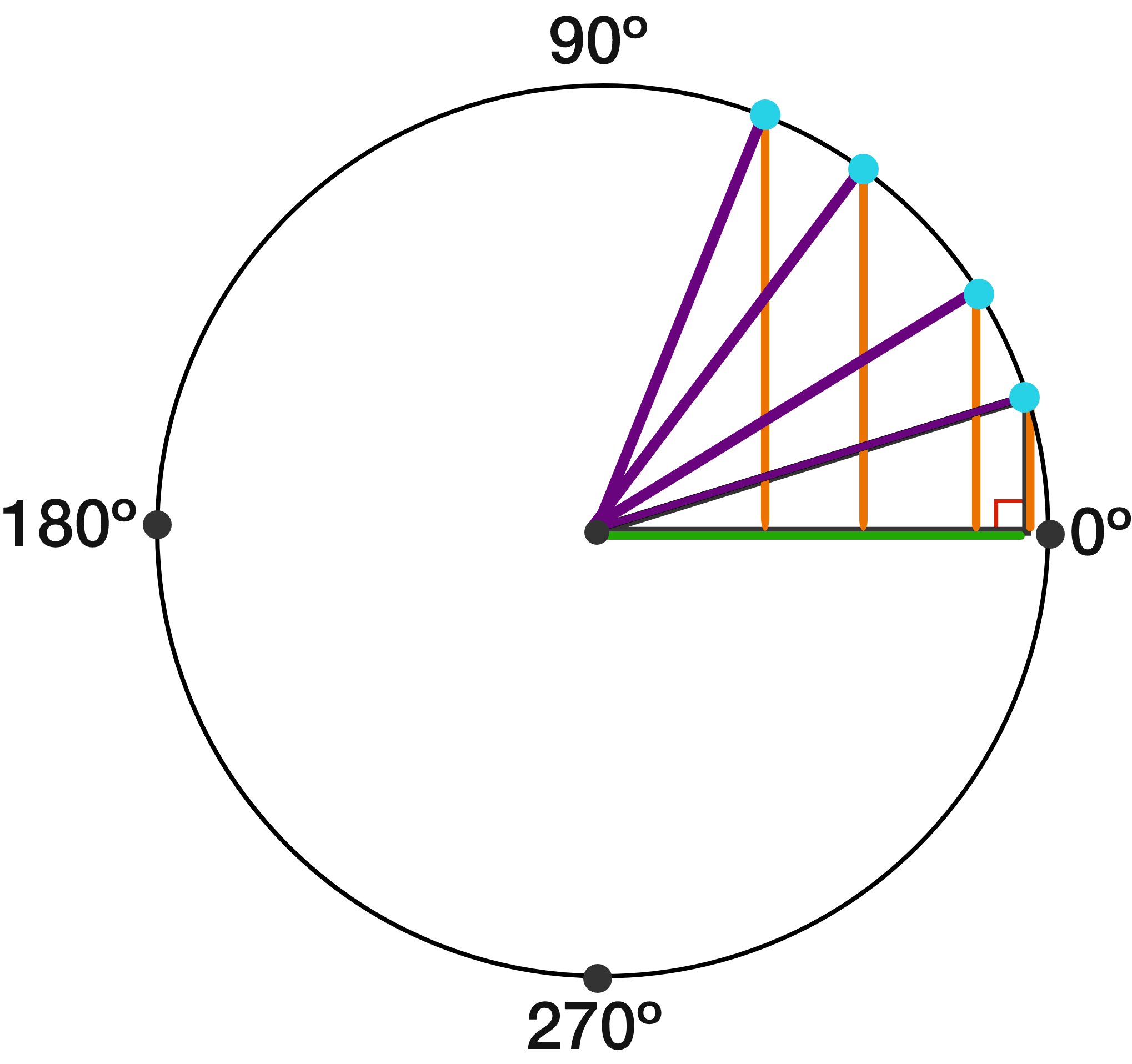As the blue point is tracing a circle, we can keep it going and trace a complete circle, from $0^\circ$ (on the right) to $90^\circ$ (on the top) all the way around back to 360 degrees (on the right again).

The advantage of this is we aren't restricted to a triangle anymore, and we can talk about things like the sine of 180 degrees.

What is the sine of 180 degrees? (Reminder: from the triangle below, $\sin(x) = \frac{O}{H} .$# Exploring the Sine

We saw in the last question that, by using a circle, we can define the sines of angles too large to exist inside of a right triangle.

In the next quiz, we'll see how we can use this reasoning to graph the sine function on a coordinate plane.

×